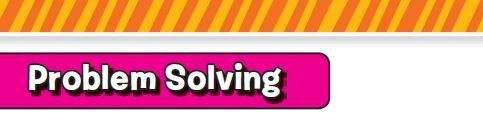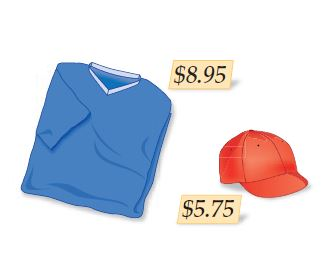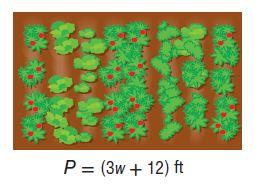Homework Explained - Math Practice 101Dear guest, you are not a registered member. As a guest, you only have read-only access to our books, tests and other practice materials.

As a registered member you can:

Registration is free and doesn't require any type of payment information. Click here to Register.
Go to page:
Chapter 5: Expressions; Chapter Review

###• Question 1

Write an expression that represents the cost in dollars of buying any number of hats h and any number of shirts s. Then find the cost of buying 3 hats and 5 shirts.•  $• Question 2 Reason Abstractly Tanya collected$4.50 for the first car washed at a band fundraiser. After the second and third cars were washed, the donations totaled $9 and$13.50, respectively. Suppose this donation pattern continues. Write the next three terms in the sequence. Then write an algebraic expression to find the amount of money earned for the number of cars washed c.
Terms:
Expression:

• Type below:
• Question 3

Graham earned 8, 13, 7, 12, and 9 points on his last few homework assignments. Use mental math to find his total number of points. Explain.

•  $$\text{points}$$
• Question 4

Carlita had some pictures printed for $0.20 per print. Find the total cost if Carlita had 65 pictures printed. Justify your answer by using the Distributive Property. • $
• Question 5

The angle measures of a triangle are $$(x - 7)^0$$, $$(x)^0$$, and $$(3x + 2)^0$$. Write an expression in simplest form to represent all of the measures of the angles of the triangle.

• Type below:
• Question 6

The perimeter of a rectangular garden is shown at the right. What is the perimeter of the garden in factored form?• Type below:

Yes, email page to my online tutor. (if you didn't add a tutor yet, you can add one here)